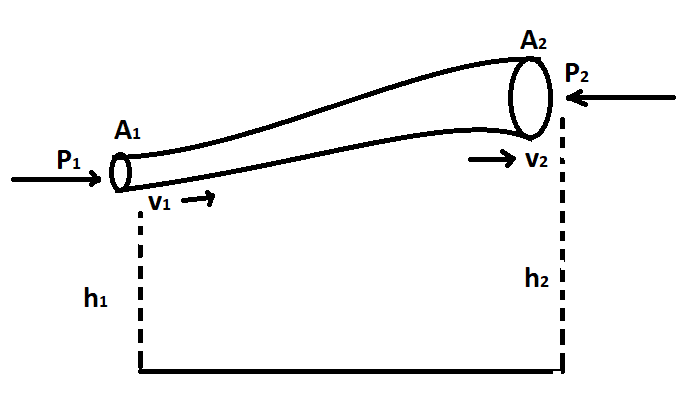# 10 Assumption of Bernoulli’s Theorem

6th July 2019

## 10 Assumption of Bernoulli’s Theorem

Following are the 10 assumptions of Bernoulli’s theorem:

1. Velocity is uniform.
2. Flow is uniform.